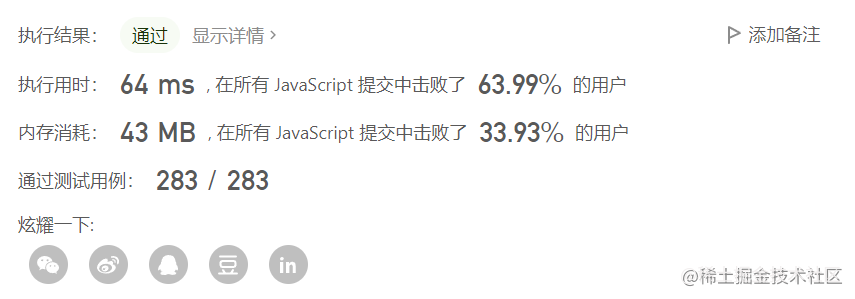# 算法简单题，吾辈重拳出击 - 爬楼梯的最少成本``````输入：cost = [10, 15, 20]

``````输入：cost = [1, 100, 1, 1, 1, 100, 1, 1, 100, 1]

## 第一反应

less[n] = Math.min(less[n-1]+cost[n-1],less[n-2]+cost[n-2])

## 第二反应

``````var minCostClimbingStairs = function(cost) {
let less = []
less=0
less=0
for(let n=2;n<cost.length;n++){
less[n] = Math.min(less[n-1]+cost[n-1],less[n-2]+cost[n-2])
}
return less[cost.length]
}## 第三反应

OK，以上便是本篇分享。点赞关注评论，为好文助力👍